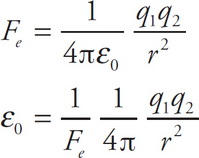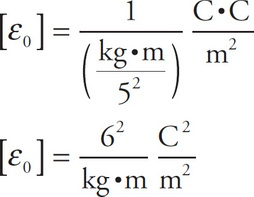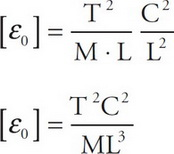# AP Physics 1 Question 49: Answer and Explanation

### Test Information

Question: 49

4. If T, C, M, and L represent the dimensions of time, charge, mass, and length, respectively, which of the following represents the correct dimensions for the permittivity of free space (ε0)?

• A. T2C2/(ML3)
• B. ML3/(T2C2)
• C. C2M/(T2L2)
• D. T2L2/(C2M)

Explanation:

A Start with the equation for electric force, and then solve for ε0:Next, substitute in the units for each variable:Finally, use the letters indicated in the problem for each unit: